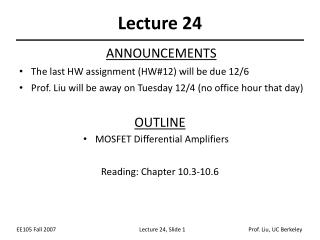Download PresentationLecture 24

# Lecture 24 - PowerPoint PPT Presentation

Lecture 24. ANNOUNCEMENTS The last HW assignment (HW#12) will be due 12/6 Prof. Liu will be away on Tuesday 12/4 (no office hour that day). OUTLINE MOSFET Differential Amplifiers Reading: Chapter 10.3-10.6. Common-Mode (CM) Response.I am the owner, or an agent authorized to act on behalf of the owner, of the copyrighted work described.
Download Presentation## Lecture 24

An Image/Link below is provided (as is) to download presentation

Download Policy: Content on the Website is provided to you AS IS for your information and personal use and may not be sold / licensed / shared on other websites without getting consent from its author.While downloading, if for some reason you are not able to download a presentation, the publisher may have deleted the file from their server.

- - - - - - - - - - - - - - - - - - - - - - - - - - E N D - - - - - - - - - - - - - - - - - - - - - - - - - -
Presentation Transcript
1. Lecture 24 ANNOUNCEMENTS • The last HW assignment (HW#12) will be due 12/6 • Prof. Liu will be away on Tuesday 12/4 (no office hour that day) OUTLINE • MOSFET Differential Amplifiers Reading: Chapter 10.3-10.6

2. Common-Mode (CM) Response • Similarly to its BJT counterpart, a MOSFET differential pair produces zero differential output as VCM changes.

3. Equilibrium Overdrive Voltage • The equilibrium overdrive voltage is defined as VGS-VTH when M1 and M2 each carry a current of ISS/2.

4. Minimum CM Output Voltage • In order to maintain M1 and M2 in saturation, the common-mode output voltage cannot fall below VCM-VTH. • This value usually limits voltage gain.

5. Differential Response

6. Small-Signal Response • For small input voltages (+DV and -DV), the gm values are ~equal, so the increase in ID1 and decrease in ID2 are ~equal in magnitude. Thus, the voltage at node P is constant and can be considered as AC ground.

7. Small-Signal Differential Gain • Since the output signal changes by -2gmVRD when the input signal changes by 2V, the small-signal voltage gain is –gmRD. • Note that the voltage gain is the same as for a CS stage, but that the power dissipation is doubled.

8. Large-Signal Analysis

9. Maximum Differential Input Voltage • There exists a finite differential input voltage that completely steers the tail current from one transistor to the other. This value is known as the maximum differential input voltage.

10. MOSFET vs. BJT Differential Pairs • In a MOSFET differential pair, there exists a finite differential input voltage to completely switch the current from one transistor to the other, whereas in a BJT differential pair that voltage is infinite. MOSFET Differential Pair BJT Differential Pair

11. Effect of Doubling the Tail Current • If ISS is doubled, the equilibrium overdrive voltage for each transistor increases by , thus Vin,max increases by as well. Moreover, the differential output swing will double.

12. Effect of Doubling W/L • If W/L is doubled, the equilibrium overdrive voltage is lowered by , thus Vin,max will be lowered by as well. The differential output swing will be unchanged.

13. Small-Signal Analysis • When the input differential signal is small compared to 4ISS/nCox(W/L), the output differential current is ~linearly proportional to it: • We can use the small-signal model to prove that the change in tail node voltage (vP) is zero:

14. Virtual Ground and Half Circuit • Since the voltage at node P does not change for small input signals, the half circuit can be used to calculate the voltage gain.

15. MOSFET Diff. Pair Frequency Response • Since the MOSFET differential pair can be analyzed using its half-circuit, its transfer function, I/O impedances, locations of poles/zeros are the same as that of the half circuit’s.

16. Example

17. Half Circuit Example 1 Half circuit for small-signal analysis

18. Half Circuit Example 2 Half circuit for small-signal analysis

19. MOSFET Cascode Differential Pair Half circuit for small-signal analysis

20. MOSFET Telescopic Cascode Amplifier Half circuit for small-signal analysis

21. CM to DM Conversion Gain, ACM-DM • If finite tail impedance and asymmetry are both present, then the differential output signal will contain a portion of the input common-mode signal.

22. MOS Diff. Pair with Active Load • Similarly to its BJT counterpart, a MOSFET differential pair can use an active load to enhance its single-ended output.

23. Asymmetric Differential Pair • Because of the vast difference in magnitude of the resistances seen at the drains of M1 and M2, the voltage swings at these two nodes are different and therefore node P cannot be viewed as a virtual ground…

24. Thevenin Equivalent of the Input Pair

25. Simplified Diff. Pair w/ Active Load